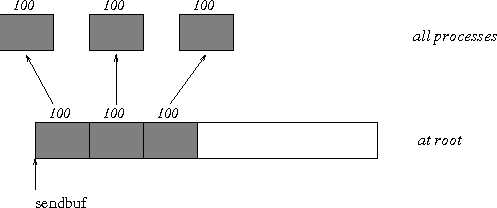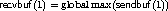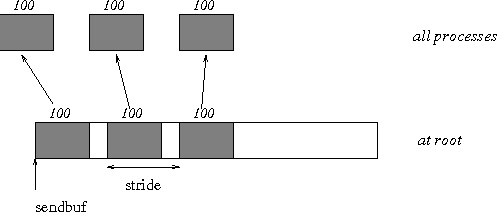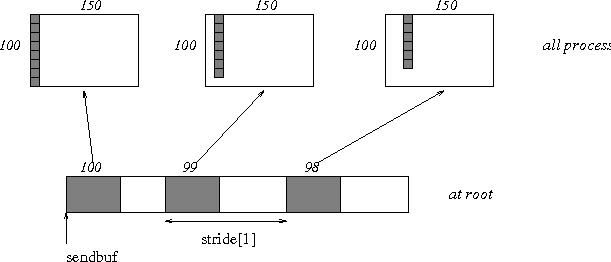## 95. Examples using MPI_SCATTER, MPI_SCATTERVUp: Scatter Next: Gather-to-all Previous: Scatter

The examples in this section use intracommunicators.
Example

The reverse of Example Examples using MPI_GATHER, MPI_GATHERV . Scatter sets of 100 ints from the root to each process in the group. See Figure 9 .

```    MPI_Comm comm;
int gsize,*sendbuf;
int root, rbuf;
...
MPI_Comm_size( comm, &gsize);
sendbuf = (int *)malloc(gsize*100*sizeof(int));
...
MPI_Scatter( sendbuf, 100, MPI_INT, rbuf, 100, MPI_INT, root, comm);
```Example

The reverse of Example Examples using MPI_GATHER, MPI_GATHERV . The root process scatters sets of 100 ints to the other processes, but the sets of 100 are stride ints apart in the sending buffer. Requires use of MPI_SCATTERV. Assume. See Figure 10 .

```    MPI_Comm comm;
int gsize,*sendbuf;
int root, rbuf, i, *displs, *scounts;

...

MPI_Comm_size( comm, &gsize);
sendbuf = (int *)malloc(gsize*stride*sizeof(int));
...
displs = (int *)malloc(gsize*sizeof(int));
scounts = (int *)malloc(gsize*sizeof(int));
for (i=0; i<gsize; ++i) {
displs[i] = i*stride;
scounts[i] = 100;
}
MPI_Scatterv( sendbuf, scounts, displs, MPI_INT, rbuf, 100, MPI_INT,
root, comm);
```Example

The reverse of Example Examples using MPI_GATHER, MPI_GATHERV . We have a varying stride between blocks at sending (root) side, at the receiving side we receive into the i-th column of a 100×150 C array. See Figure 11 .

```    MPI_Comm comm;
int gsize,recvarray,*rptr;
int root, *sendbuf, myrank, bufsize, *stride;
MPI_Datatype rtype;
int i, *displs, *scounts, offset;
...
MPI_Comm_size( comm, &gsize);
MPI_Comm_rank( comm, &myrank );

stride = (int *)malloc(gsize*sizeof(int));
...
/* stride[i] for i = 0 to gsize-1 is set somehow
* sendbuf comes from elsewhere
*/
...
displs = (int *)malloc(gsize*sizeof(int));
scounts = (int *)malloc(gsize*sizeof(int));
offset = 0;
for (i=0; i<gsize; ++i) {
displs[i] = offset;
offset += stride[i];
scounts[i] = 100 - i;
}
/* Create datatype for the column we are receiving
*/
MPI_Type_vector( 100-myrank, 1, 150, MPI_INT, &rtype);
MPI_Type_commit( &rtype );
rptr = &recvarray[myrank];
MPI_Scatterv( sendbuf, scounts, displs, MPI_INT, rptr, 1, rtype,
root, comm);

```Up: Scatter Next: Gather-to-all Previous: Scatter